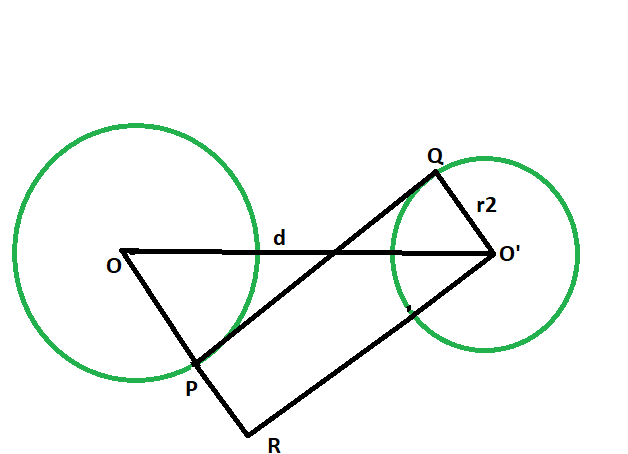Related Articles

# Length of the transverse common tangent between the two non intersecting circles

• Last Updated : 15 Mar, 2021

Given two circles of given radii, having there centres a given distance apart, such that the circles don’t touch each other. The task is to find the length of the transverse common tangent between the circles.
Examples:

```Input: r1 = 4, r2 = 6, d = 12
Output: 6.63325

Input: r1 = 7, r2 = 9, d = 21
Output: 13.6015```Approach

1. Let the radii of the circles be r1 & r2 respectively.
2. Let the distance between the centers be d units.
3. Draw a line O’R parallel to PQ,
4. angle OPQ = angle RPQ = 90 deg
angle O’QP = 90 deg
{ line joining the center of the circle to the point of contact makes an angle of 90 degree with the tangent }

5. angle RPQ + angle O’QP = 180 deg
PR || O’Q
6. Since opposite sides are parallel and interior angles are 90, therefore O’PQR is a rectangle.
7. O’Q = RP = r2 and PQ = O’R
8. In triangle OO’R
angle ORO’ = 90 deg
By Pythagoras theorem
OR^2 + O’R^2 = OO’^2
O’R^2 = OO’^2 – OR^2
O’R^2 = d^2 – (r1+r2)^2
O’R^2 = √(d^2 – (r1+r2)^2)## C++

 `// C++ program to find the length``// of the transverse common tangent``// between two circles which``// do not touch each other` `#include ``using` `namespace` `std;` `// Function to find the length``// of the transverse common tangent``void` `lengthOfTangent(``double` `r1, ``double` `r2, ``double` `d)``{` `    ``cout << ``"The length of the transverse"``         ``<< ``" common tangent is "``         ``<< ``sqrt``(``pow``(d, 2) - ``pow``((r1 + r2), 2))``         ``<< endl;``}` `// Driver code``int` `main()``{``    ``double` `r1 = 4, r2 = 6, d = 12;``    ``lengthOfTangent(r1, r2, d);``    ``return` `0;``}`

## Java

 `// Java program to find the length``// of the transverse common tangent``// between two circles which``// do not touch each other``class` `GFG {` `    ``// Function to find the length``    ``// of the transverse common tangent``    ``static` `void` `lengthOfTangent(``double` `r1,``                                ``double` `r2, ``double` `d)``    ``{` `        ``System.out.println(``"The length of the transverse"``                           ``+ ``" common tangent is "``                           ``+ Math.sqrt(Math.pow(d, ``2``)``                                       ``- Math.pow((r1 + r2), ``2``)));``    ``}` `    ``// Driver code``    ``public` `static` `void` `main(String args[])``    ``{``        ``double` `r1 = ``4``, r2 = ``6``, d = ``12``;``        ``lengthOfTangent(r1, r2, d);``    ``}``}` `// This code has been contributed by 29AjayKumar`

## Python3

 `# python 3 program to find the length``# of the transverse common tangent``# between two circles which``# do not touch each other``from` `math ``import` `sqrt, ``pow` `# Function to find the length``# of the transverse common tangent``def` `lengthOfTangent(r1, r2, d):``    ``print``(``"The length of the transverse"``,``                     ``"common tangent is"``,``          ``'{0:.6g}'``.``format``(sqrt(``pow``(d, ``2``) ``-``                                ``pow``((r1 ``+` `r2), ``2``))))` `# Driver code``if` `__name__ ``=``=` `'__main__'``:``    ``r1 ``=` `4``    ``r2 ``=` `6``    ``d ``=` `12``    ``lengthOfTangent(r1, r2, d)``    ` `# This code is contributed by``# Surendra_Gangwar`

## C#

 `// C# program to find the length``// of the transverse common tangent``// between two circles which``// do not touch each other``using` `System;` `class` `GFG {``    ``// Function to find the length``    ``// of the transverse common tangent``    ``static` `void` `lengthOfTangent(``double` `r1,``                                ``double` `r2, ``double` `d)``    ``{` `        ``Console.WriteLine(``"The length of the transverse"``                          ``+ ``" common tangent is "``                          ``+ Math.Sqrt(Math.Pow(d, 2)``                                      ``- Math.Pow((r1 + r2), 2)));``    ``}` `    ``// Driver code``    ``static` `public` `void` `Main()``    ``{``        ``double` `r1 = 4, r2 = 6, d = 12;``        ``lengthOfTangent(r1, r2, d);``    ``}``}` `// This code has been contributed by ajit.`

## PHP

 ``

## Javascript

 ``
Output:
`The length of the transverse common tangent is 6.63325`

Attention reader! Don’t stop learning now. Get hold of all the important DSA concepts with the DSA Self Paced Course at a student-friendly price and become industry ready.  To complete your preparation from learning a language to DS Algo and many more,  please refer Complete Interview Preparation Course.

In case you wish to attend live classes with experts, please refer DSA Live Classes for Working Professionals and Competitive Programming Live for Students.

My Personal Notes arrow_drop_up